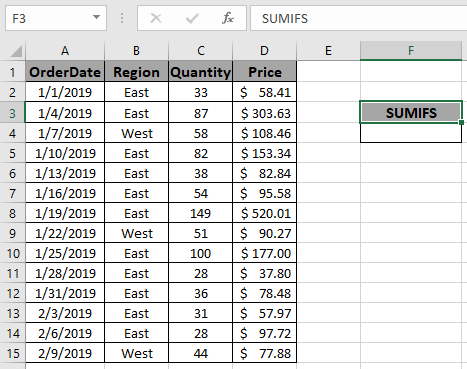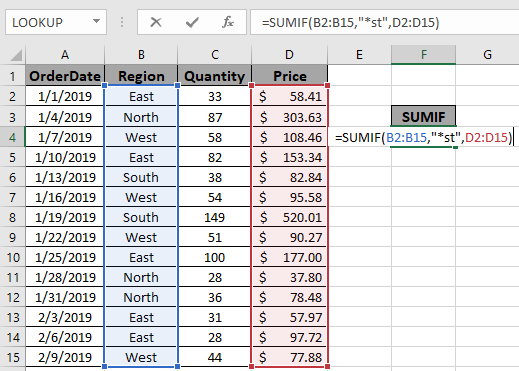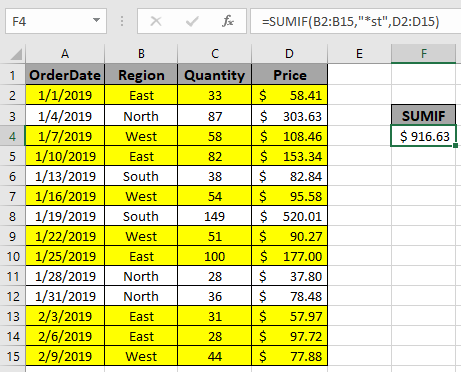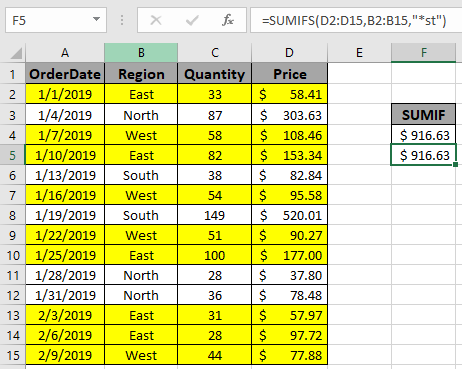# How to SUM If Ends With

In this article, we will learn how to sum if values only if text ends with a certain characters.

Sometimes we need to find the sum of values with matching end value. SUMIF function can sum up the array where the criteria range contains text that ends with a particular sub-string. We will use wildcards in the Excel SUMIF function to pull this off.

Excel lets you use Wildcard characters for catching strings and perform functions on it. Here we just need to use one type of wildcard as an example.

1. Asterisk (*): This wildcard is used to find any number of characters preceding or following any character.

We will construct a formula out of it. First, the text value that needs to be matched is combined with wildcard to search values matching the same. Then the matched values will return values corresponding to the same. Last but not least, the SUM operator will return the sum of the values.
Use the formula

= SUMIF ( range , "*value" , [sum_range] )

Explanation:

1. "*value" matches the value which ends with the value.
2. The value is matched with values in the range array.
3. Corresponding values add up to return the sum of the values matching the value.

Let's get this through with an example.

Here we have a list of received orders and their respective values.Here we need to get the sum if the region is either East or West. Since both string contains "st" at the end, we can use the * wildcard instead of SUMIF with or logic.

We will use the formula to get the total amount.

= SUMIF ( B2:B15 , "*st" , D2:D15 )

Explanation for the formula:

D2:D15 : Sum_range where values gets sum

B2:B15 :  range where criteria *value (criteria) is matched.

"*st" : value criteria, matches East or West.Here the argument arrays to the function is given as cell reference.

Use the formula as stated above to calculate the total price and click Enter.As you can see the formula returns \$916.63 , the Total price for the yellow rows marked.

You can also use the SUMIFS function formula to get the same result.
SUMIFS function returns the sum of the range having multiple criteria.
Syntax of the formula:

= SUMIFS ( Sum_range , range1 , "*value",... )These both function can handle wildcards. And hence the result.

Notes:

1. The SUMIFS function supports logical operators like <, >, <>, = but these are used using double quote sign ( " ) .
2. The SUMIFS function supports Wildcards ( * , ? ) which helps in extracting values by having phrases.
3. Non - numeric values must be provided in double quotes ("*value").
4. See the whole list as the function returns values wherever matches.
5. The array of arguments to the function can be given as cell reference or named ranges.
6. The function returns the sum of the values satisfying all the conditions.

Hope you understood how to get the SUM values if matches end value in Excel. Explore more articles on Excel SUMIFS function here. Please feel free to state your query or feedback for the above article.

Related Articles

How to Use SUMIF Function in Excel

How to Use SUMIFS Function in Excel

Sum if cell contains text in other cell in Excel

SUM if value is less than

Sum if date is greater than given date

Sum if cell is equal to value

Sum if cell is not equal to value

SUM if date is between

Sum if cells contain specific text

Sum If Greater Than 0 in Excel

3 SUMIF with Or Formulas

Excel SUMIF Not Blank Cells

SUMIFS using AND-OR logic

SUMIF with non-blank cells

SUMIFS with dates in Excel

Popular Articles

50 Excel Shortcut to Increase Your Productivity

Edit a dropdown list

Absolute reference in Excel

If with conditional formatting

If with wildcards

Vlookup by date

Convert Inches To Feet and Inches in Excel 2016

Join first and last name in excel

Count cells which match either A or B

Terms and Conditions of use

The applications/code on this site are distributed as is and without warranties or liability. In no event shall the owner of the copyrights, or the authors of the applications/code be liable for any loss of profit, any problems or any damage resulting from the use or evaluation of the applications/code.